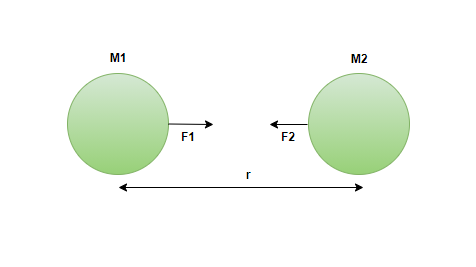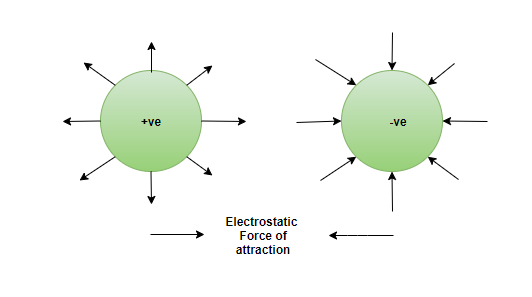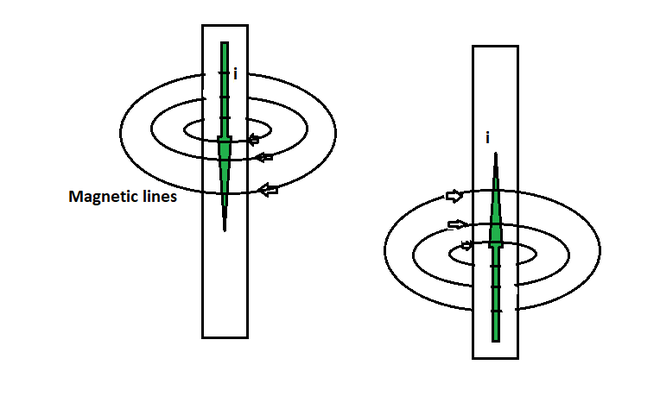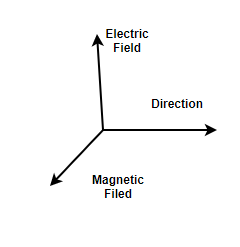Open in App
Not now

# Fundamental Forces

• Last Updated : 26 May, 2021

The most beautiful phenomenon that can be seen in physics is that how universe is so disciplined and synced together. The force has kept the universe bind together. Forces have always played an important role in the human existence, but it is not realized by humans. Human beings constantly experience some force acting on them like gravitational force, etc.  There are some forces that naturally exist in the universe, they are known as Fundamental Forces. Let’s learn about them in some detail.

### Force

Force is an interaction, either with contact or without contact. When there is no opposition given to the Force, It can result in changing the motion, shape, position, of a body. Whenever some interaction occurs between two objects, there is a certain force acting upon them. There are many types of Forces present, For instance, Applied Force, Gravitational Force, Frictional Force, Tension Force, etc.

### Fundamental Forces in nature

These forces are so well-defined that they cover the macroscopic and microscopic forces present in nature. The forces learned or seen by humans in everyday life, from frictional force, pull, push, thrust, etc. are known as derived forces, and they are not considered the fundamental forces. The derived forces are actually derived from the fundamental forces in some way or the other. Some Fundamental Forces are,

• Gravitational Force
• Electromagnetic Force
• Strong Nuclear Force
• Weak Nuclear Force

These above-mentioned forces are responsible for all the observations obtained in forces present in the nature.

Gravitational Force

This force exists by the virtue of the masses of any objects. Gravitational force is the mutual force of attraction between two masses. Attraction between any mass and Earth is called Gravity. Isaac Newton first gave the concept of gravity. Gravity is the most intuitive and the weakest force present in nature. The Irony is that Gravity actually holds the planet, Solar system, Entire Universe together, yet it is known to be the weakest force present in nature.

The Force of Gravitation is given as,Formula for Gravitational Force,Where, F = Force of Gravitation

M1, M2 = Masses 1 and 2

r = Distance between the masses

G = Gravitational Constant (6.67× 10-11m3kg-1s-2)

Electromagnetic Force

Charges when they are at rest exert a force at each other known as the Electric force of attraction/repulsion. Like charges repel each other while unlike charges attract each other. When charges start to move and become dynamic, they develop magnetic field lines around and have magnetic force, these two forces combined are known as Electromagnetic forces present in nature.Magnetic force produced by current (moving charge)

Combining the above two forces which are always perpendicular to each other will give rise to Electromagnetic Force. The force is produced by massless bosons and photons present in the charges, Electromagnetic force exists in nature and is responsible for many derived forces we experience every day, for example, Friction Force, Normal Force, Elasticity, and so on.Strong Nuclear Force

It is the force of attraction between Protons and Neutrons. The force is the same whether protons and protons are present or neutrons and neutrons are present or protons and neutrons are looked at. In short, a Strong nuclear force exists between all nucleons. In short Ranges, this Force is the strongest among all the forces. It is important to note that at a distance of 10-13 cm, this force vanishes.

Weak Nuclear Force

This Force is seen In the β Decay of a nucleus. Scientist named Wolfgang Pauli First predicted a particle named Neutrino. The Neutrino is an uncharged Particle that is released along with the electron in the β Decay process. During β Decay, when Beta Particle is ejected from an Atom, it tends to accelerate away from the atom and some force is required to accelerate the particle known as Weak Nuclear Force. Weak Nuclear Force is stronger than gravitational force but weaker than a strong nuclear force.

Table to represent Range and Relative Strength of Different Forces:

### Conceptual Questions

Question 1: Which Fundamental Force is the strongest and weakest among the fundamental forces present in nature?

The strongest force present in nature is the Strong Nuclear Force and the weakest force present in nature at atomic scale is Gravity.

Question 2: Which of the following forces are Fundamental, and which are derived in nature?

Weak Nuclear Force, Friction Force, Gravity, Elasticity, Electromagnetic Force, Push

Fundamental Forces ⇢ Weak Nuclear Force, Gravity, Electromagnetic Force.

Derived Force ⇢ Friction Force, Elasticity, Push.

Question 3: A statement is being used now “Gravity is not a force” Throw light on this statement.

Isaac Newton initially discovered Gravity and Gravitational Force. It was then stated that gravity is a Force. Later on, with the help of Theory of Relativity, Einstein stated that Gravity is actually not a force but a result of space-time orientation. It is a consequence of masses moving along a geodesic lines in space time.

Question 4: If the masses of 2 objects are doubled and the space between them is also doubled. How will the gravitational Force between them change?

Gravitational Force is given as,When, Mass 1 and Mass 2 is doubled, M1’=2M1, M2’=2M2

Distance between the masses is doubled, r’= 2r

New Gravitational Force Between them,Hence, The new value of gravitational Force will be same as the old Gravitational Force.

Question 5: What are Pseudo Forces? Give Examples.

Pseudo Forces are also known as Inertial Force or Fictitious Force. These forces actually do not exist and are the apparent forces that are seen due to fact that they are defined from a non-inertial frame.

Example: A man sees another man going in a car and realized that some force is acting on the man in the car. This type of force seen is real as it is seen from a non-accelerated or inertial frame. However, the man in the car if looks at the man standing would feel that some force is acted upon the man and he is going backwards, this force is pseudo force, Since this force is seen from a non-inertial frame or an accelerated frame.

Question 6: Which two forces have infinite range?